# Acute angle

Here is everything you need to know about an acute angle, including what it is and how to identify it.

Students first learn about acute angles in 4 th grade with their work in geometric measurements. They expand that knowledge as they progress through middle school.

## What is an acute angle?

An acute angle is an angle that is less than 90^{\circ} and greater than 0^{\circ} .

All acute angles fall
somewhere in between
these two angles.

Acute angles can be formed when two rays extend from a common point.

For example,

The symbol (\angle) is used to name an angle. The angle can be named after its vertex or the vertex and a point on each ray.

The acute angle above can be named \angle \mathrm{F}, \angle \mathrm{CFT} \text { or } \angle \mathrm{TFC} .

If you picture a \, 90^{\circ} angle (shown in blue), it is clear that \angle \mathrm{CFT} \, is less than \, 90^{\circ} , but more than 0^{\circ} . Visualizing a \, 90^{\circ} angle lets you identify most acute angles, but when in doubt, measure the angle with a protractor to prove that an angle is acute.

Acute angles are also formed when the sides of 2D shapes (polygons) come together.

For example,

This triangle has all acute interior angles.

Some shapes have some acute angles, but also other types of angles.

For example,

Both the triangle and the trapezoid have two acute angles, but also other angles.

Acute angles can also be formed when two straight lines cross.

For example,

Two acute angles are formed when these lines cross, but there are also other angles.

There will always be two acute angles if the straight lines are not perpendicular (at 90^\circ).

### What is an acute angle?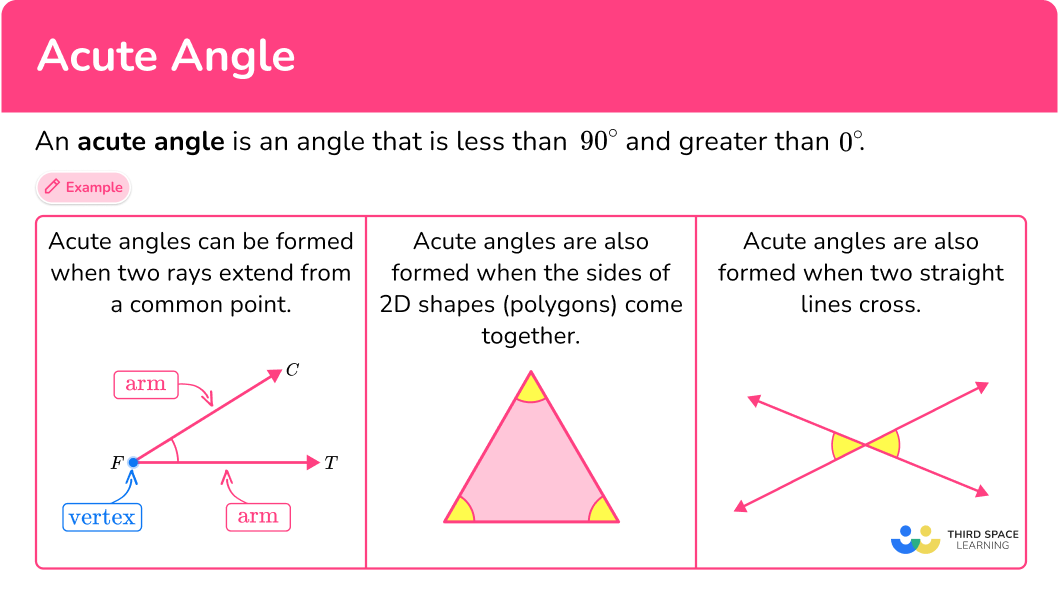## Common Core State Standards

How does this relate to 4 th grade math?

• Grade 4 – Measurement and Data (4.MD.C.5a)
An angle is measured with reference to a circle with its center at the common endpoint of the rays, by considering the fraction of the circular arc between the points where the two rays intersect the circle. An angle that turns through \cfrac{1}{360} \, of a circle is called a “one-degree angle,” and can be used to measure angles.

• Grade 4 – Measurement and Data (4.MD.C.5b)
An angle that turns through n one-degree angles is said to have an angle measure of n degrees.

• Grade 4 – Geometry (4.G.A.1)
Draw points, lines, line segments, rays, angles (right, acute, obtuse), and perpendicular and parallel lines. Identify these in two-dimensional figures.

## How to identify an acute angle

In order to identify an acute angle:

1. Recall the definition of an acute angle.
2. Explain whether or not the angle is acute.

## Acute angle examples

### Example 1: classify a given angle

Is the angle an acute angle?

1. Recall the definition of an acute angle.

An acute angle is less than 90^{\circ} and greater than 0^{\circ} .

2Explain whether or not the angle is acute.

Comparing the angle to a \, 90^{\circ} angle (in blue), the angle is an acute angle, because it is less than \, 90^{\circ} and greater than 0^{\circ} .

### Example 2: classify a given angle

Is the angle an acute angle?

An acute angle is less than 90^{\circ} and greater than 0^{\circ} .

Comparing the angle to a \, 90^{\circ} angle (in blue), the angle is NOT an acute angle, because it is greater than 90^{\circ} .

### Example 3: identify acute angles in a given shape

How many acute angles does this shape have?

An acute angle is less than 90^{\circ} and greater than 0^{\circ} .

Comparing the angle to a \, 90^{\circ} angle (in blue), the bottom left angle and the top right angle are acute angles, because they are less than 90^{\circ} and greater than 0^{\circ} .

Comparing the angle to a 90^{\circ} angle (in blue), the bottom right angle and the top left angle are NOT acute angles, because they are greater than 90^{\circ} .

This shape (parallelogram) has 2 acute angles.

### Example 4: identify acute angles in a given shape

How many acute angles does this regular hexagon have?

An acute angle is less than 90^{\circ} and greater than 0^{\circ} .

Comparing the angle to a \, 90^{\circ} angle (in blue), the bottom left angle is NOT an acute angle, because it is greater than 90^{\circ} .

Since the hexagon is regular, all angles are congruent, so this regular hexagon has no acute angles.

### Example 5: identify acute angles in intersecting lines

How many acute angles are formed by these crossing lines?

An acute angle is less than 90^{\circ} and greater than 0^{\circ} .

There are four angles formed by the crossing lines. The left and right angles are clearly less than 90^{\circ} and greater than 0^{\circ} .

The top and bottom angles are clearly greater than 90^{\circ} .

There are 2 acute angles formed by these crossing lines.

### Example 6: identify real-life examples of acute angles

How many acute angles do you see in the swing set?

An acute angle is less than 90^{\circ} and greater than 0^{\circ} .

There are two angles formed by the legs of the swing set. They are clearly less than 90^{\circ} and greater than 0^{\circ} .

There are two angles formed by the top of the swing set meeting a leg. They are clearly less than 90^{\circ} and greater than 0^{\circ} .

There are 4 acute angles in the swing set.

### Teaching tips for an acute angle

• When learning about angles, give students plenty of practice drawing and identifying their own acute angles. Always encourage students to justify their classification of an acute angle by comparing it to a \, 90^{\circ} or \, 0^{\circ} angle or by measuring with a protractor.

• Give students opportunities to use digital platforms that let students create or classify angles. This allows students to easily create angles and practice identifying them in greater volume than drawing them provides. Both types of practice are important, but digital platforms can be especially useful for students who have trouble drawing angles or for whom drawing angles is very time consuming.

• When teaching students to identify angles on a computer or piece of paper where no protractor is given, students can use a notecard or corner of a piece of paper to compare the angle to a right angle.

• Worksheets can provide useful acute angle practice, but be sure to look for ones that show acute angles in a variety of ways (angle diagrams, shapes, real world pictures, line diagrams) and orientations, so students learn to think flexibly about angles.

• Instead of giving students study materials and asking them to memorize the definition, challenge students to look for and record acute angles they see in the real world.

### Easy mistakes to make

• Confusing acute angles with other types of angles
There are other angles besides acute: right angles are \, 90^{\circ} , obtuse angles are between \, 90^{\circ} and \, 180^{\circ} , straight angles are 180^{\circ} and reflex angles are between 180^{\circ} and \, 360^{\circ} . Without repeated exposure or practice, it is easy to get these confused.

• Not recognizing an acute angle that has an uncommon orientation
Acute angles can be in any orientation.
For example,
All the angles below are acute, no matter how they are turned.

• Making incorrect assumptions about angles close to 90^{\circ}
It can be hard to classify by just looking at angle measures that are close to 90^{\circ} (like 89^{\circ} or \, 91^{\circ} ). When in doubt, always measure with a protractor.
For example,
All the angles below are too close to tell and should be measured with a protractor to verify what type of angle they are.

• Thinking all angles of a triangle are acute
While all triangles have at least \, 2 acute angles, they do not all have to be acute. Specifically in obtuse triangles and right triangles.
For example,

• Forgetting the degree sign
When measuring or estimating angles, your answer must always be given in degrees.
For example,
Record an angle measure as 45^{\circ} , not \, 45 .

### Acute angle practice questions

1) Is the angle acute? Why or why not?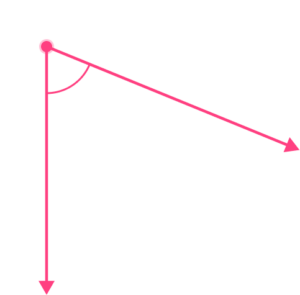Yes, because it is less than 90^{\circ} and greater than \, 0^{\circ}No, because it is less than \, 90^{\circ} and greater than \, 0^{\circ}Yes, because it is greater than \, 90^{\circ}No, because it is greater than \, 90^{\circ}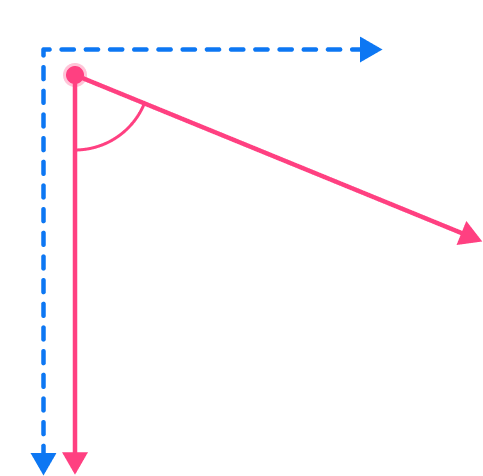Comparing the angle to a \, 90^{\circ} angle (in blue), the angle is an acute angle, because it is less than \, 90^{\circ} and greater than \, 0^{\circ} .

2) Is the angle acute? Why or why not?Yes, because it is less than   90^{\circ} and greater than \, 0^{\circ}No, because it is less than \, 90^{\circ} and greater than \, 0^{\circ}Yes, because it is greater than \, 90^{\circ}No, because it is greater than \, 90^{\circ}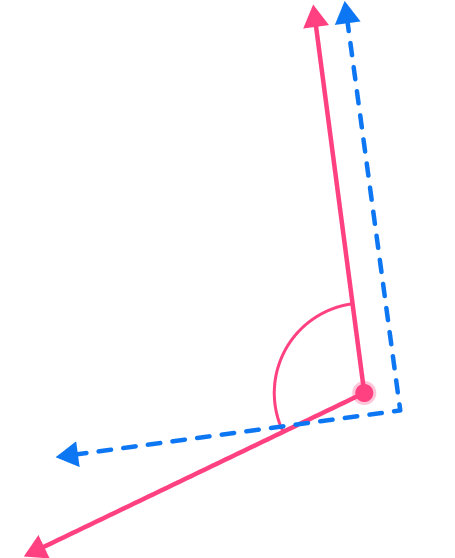Comparing the angle to a \, 90^{\circ} angle (in blue), the angle is NOT an acute angle, because it is greater than \, 90^{\circ} .

3) How many acute angles does the shape have?2345Comparing the angle to a \, 90^{\circ} angle (in blue), these four angles are acute angles, because they are less than \, 90^{\circ} and greater than 0^{\circ} .These two angles are NOT acute angles, because they are greater than 90^{\circ} .

This shape (irregular hexagon) has 4 acute angles.

4) Which shape has 3 acute angles?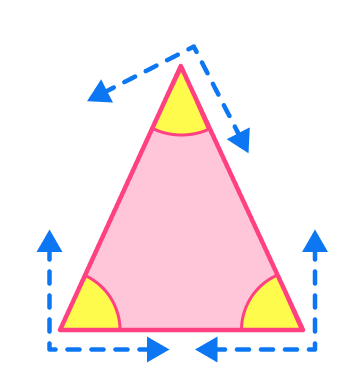Comparing the angle to a 90^{\circ} angle (in blue), these three angles are acute angles, because they are less than 90^{\circ} and greater than 0^{\circ} .

Note, because all angles in the triangle are acute, this is an acute triangle.

5) Which angles are acute?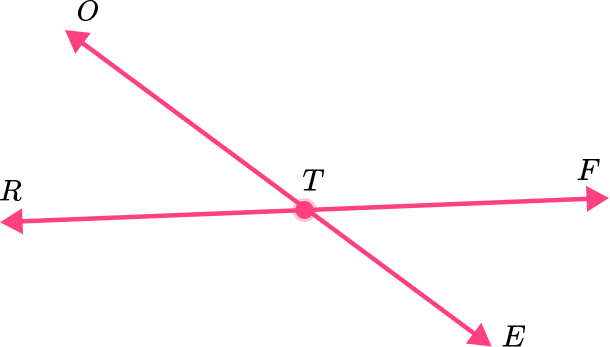\angle R T E and \angle E T F\angle E T F and \angle F T O\angle R T E and \angle F T O\angle E T F and \angle R T O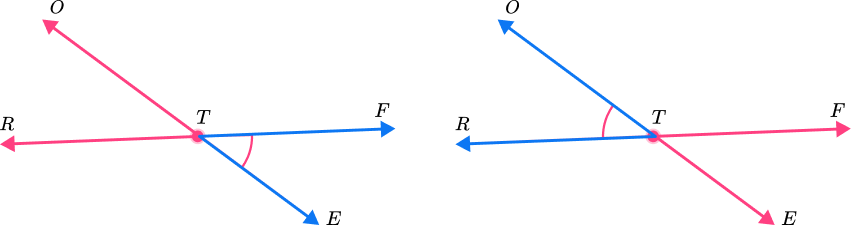These two angles ( \angle E T F and \angle R T O ) are less than 90^{\circ} and greater than 0^{\circ} , so they are acute angles.

6) How many acute angles does the game spinner have?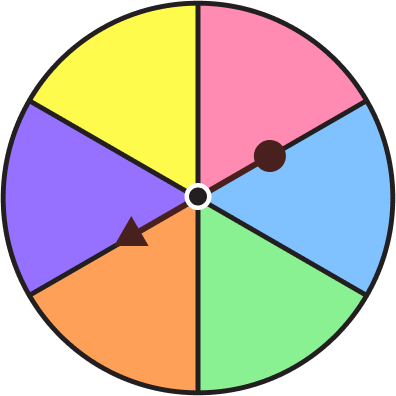31206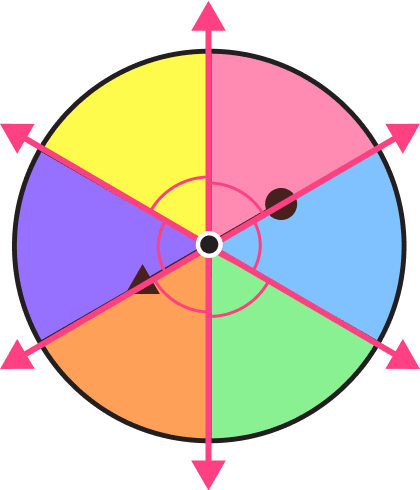There are six angles formed by the colored sections of the game spinner. They are clearly less than 90^{\circ} and greater than 0^{\circ} .

There are 6 acute angles in the game spinner.

## Acute angle FAQs

Will an equilateral triangle always have \bf{3} acute angles?

Yes, because the angles in an equilateral triangle are equal, they will always be 60 degrees and therefore acute.

Will an isosceles triangle always have \bf{3} acute angles?

No, while it can have 3 acute angles, there are right isosceles and obtuse isosceles triangles that only have 2 acute angles.

How are internal angles measured?

All angles (whether interior or exterior) can be measured in degrees and radians.

## Still stuck?

At Third Space Learning, we specialize in helping teachers and school leaders to provide personalized math support for more of their students through high-quality, online one-on-one math tutoring delivered by subject experts.

Each week, our tutors support thousands of students who are at risk of not meeting their grade-level expectations, and help accelerate their progress and boost their confidence.